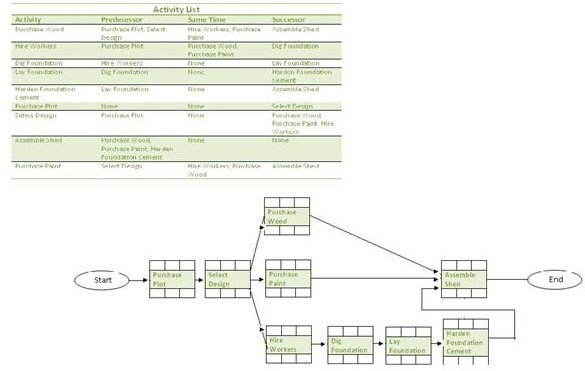# What Are the Similarities and Differences Between PERT and CPM Networks?

Page content

## For Planning and Scheduling

Both the Critical Path Method (CPM) and PERT Network are used as planning, scheduling, and controlling techniques. In many ways, they are similar. For example, both techniques were developed independently at about the same time - in 1957. The Critical Path Network or Method came out of Du Pont, while the PERT Network was a result of a project at the U.S. Navy. But that’s history, let’s dig straight into the similarities and differences between PERT and CPM networks. [caption id=“attachment_133050” align=“aligncenter” width=“600”]For accurate project scheduling, the critical path must be known[/caption]

## Similarities

The steps to create both networks are similar. The steps are:

1. Create an activity list: They are the list of activities within the project that need to be completed so that the project can be closed. The image below illustrates an activity list.

2. Create a Precedence Diagram: This is a project network diagram that illustrates the activity flow in the project. The dependencies between each activity are clearly identified. The image below illustrates a Precedence diagram.

[caption id="" align=“alignnone” width=“600”]Activities list and precedence diagram[/caption]

3. Assign estimates for each activity: The estimated time to complete each activity.

4. Identify the critical path: The longest activity execution path within the network diagram.

5. Calculate the float of each activity: The slack of each activity in the project network diagram.

Those of you who have read the series of articles on the Critical Path Method will know that these steps are typically followed while using the Critical Path Method. The key difference lies in Step 3 (Assign estimates for each activity).

## Differences Between PERT and CPM

Even if you use Estimation Best Practices, it is difficult to make estimates stick. In the Critical Path Network, a deterministic approach to estimation is used. There is no range or probability that comes into play, i.e., in the Critical Path Method, completion time variance is not accounted for. The PERT Network acknowledges that there will be a time variance (due to uncertainty) in the completion of each activity and therefore the PERT Network uses a probabilistic approach to estimating for each activity. By doing this, the completion time variance is accounted for. To estimate for an activity, the following formula is used:

Expected time = ( Optimistic + 4 x Most likely + Pessimistic ) / 6

Where,

• Optimistic: The lowest probability (Approximately 1%) that the activity will be completed within the optimistic time.
• Most likely: The highest probability of completing the activity in this time.
• Pessimistic: The longest possible time that an activity might require to get completed.

Another difference is that you can use the Critical Path Network to control both the time and the cost of the project. PERT in its pure form cannot be used for the time/cost tradeoff analysis.

## When to Use CPM and PERT

As the Critical Path Network does not account for uncertainty, it is best used in projects where the activity time estimate can be predicted fairly accurately. For example, for repetitive projects you can estimate quite accurately the time for each activity from past experience. For projects that have a higher degree of uncertainty, use the PERT Network. Most software projects will require you to account for a high-degree of uncertainty. Another useful estimation technique is the Delphi Estimation.